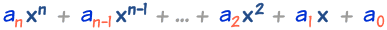# General Form of a Polynomial

A polynomial with one variable looks like this:example of a polynomial this one has 3 terms

But how do we talk about general polynomials? Ones that may have lots of terms?

## General Form

A general polynomial (of one variable) could have any number of terms:

Degree 2 (Quadratic) can have letters a,b,c:ax2 + bx + c
Degree 3 (Cubic) can have letters a,b,c,d:ax3 + bx2 + cx + d
......
But for Degree "n" letters won't work:axn + bxn-1 + ... + ?x + ?

The trouble is, we don't know what letters to end on!

 So instead of "a, b, c, ..." we use the letter "a" with a little number next to it, which says which term it belongs to:So for the general case, we use this style:And now we can say:

• an is the coefficient (the number we multiply by) for xn,
• an-1 is the coefficient for xn-1,
• ... etc, down to ...
• a1 which is the coefficient for x (because x1 = x), and
• a0 which is the constant term (because x0 = 1).

### Example: 9x4 + 5x2 - x + 7

• a4 = 9
• a3 = 0 (there is no x3 term)
• a2 = 5
• a1 = -1
• a0 = 7

Note also:

• The Degree of the polynomial is n
• an is the coefficient of the highest term xn
• an is not equal to zero (otherwise no xn term)
• an is always a Real Number
• n can be 0, 1, 2, and so on, but not infinity